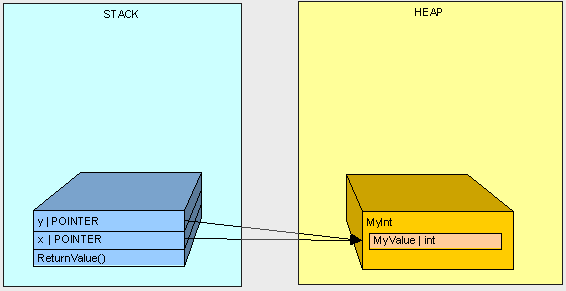C# 堆（Heap） Vs 栈 (Stack) in .NET (1/4)

.NET framework使我们不需要刻意关心内存管理和垃圾回收（GC），但是当我们需要优化应用的性能的时，我们就需要对他们有所了解。理解内存解能帮助我们知道我们所写编码中变量的行为，在这篇文章中我将描述 堆和栈的基本知识, 变量和变量的工作原理。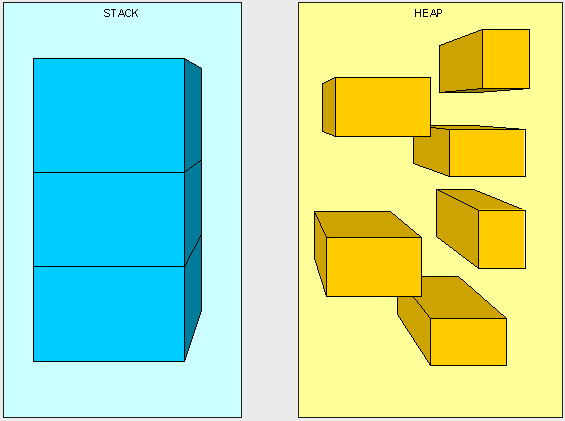• bool
• byte
• char
• decimal
• double
• enum
• float
• int
• long
• sbyte
• short
• struct
• uint
• ulong
• ushort

• class
• interface
• delegate
• object
• string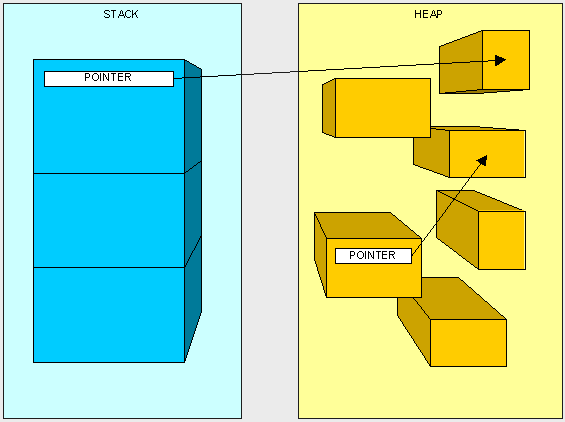1，引用类型肯定是放在堆上， 简单吧？:)
2，值类型和指针类型由他们在哪里声明决定，这个有点复杂，我们需要理解栈是怎么工作的，然后才能指出他们是在哪里声明的。

1 public int AddFive(int pValue)
2 {
3 int result;
4 result = pValue + 5;
5 return result;
6 }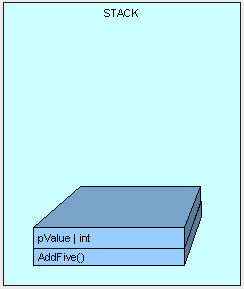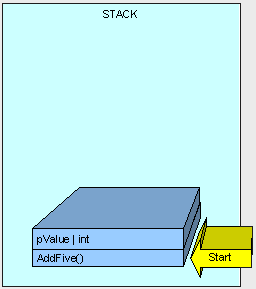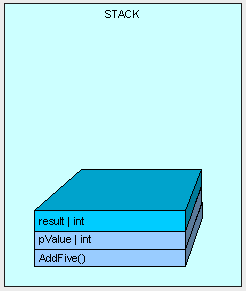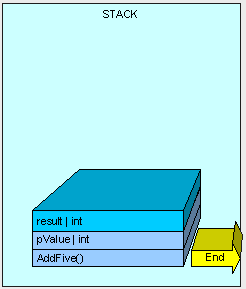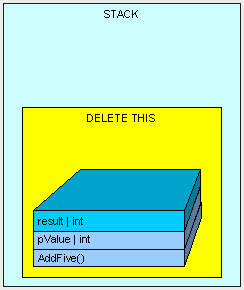1 public class MyInt
2 {
3 public int MyValue;
4 }

1 public MyInt AddFive(int pValue)
2 {
3 MyInt result = new MyInt();
4 result.MyValue = pValue + 5;
5 return result;
6 }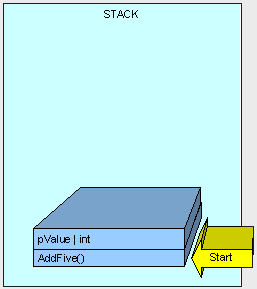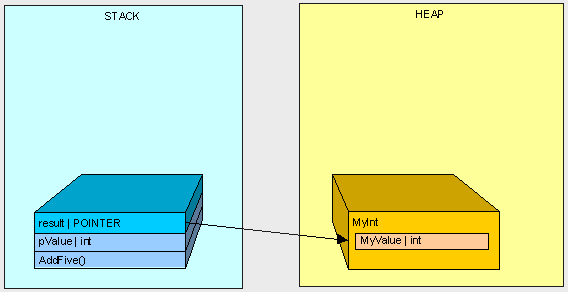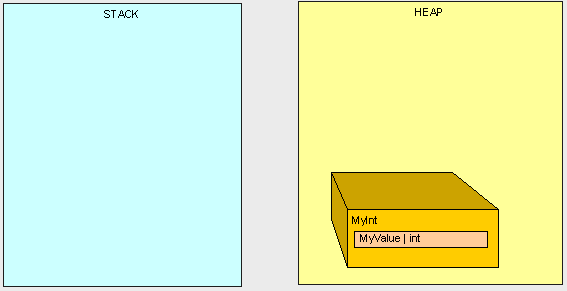1 public int ReturnValue()
2 {
3 int x = new int();
4 x = 3;
5 int y = new int();
6 y = x;
7 y = 4;
8 return x;
9 }

1 public int ReturnValue2()
2 {
3 MyInt x = new MyInt();
4 x.MyValue = 3;
5 MyInt y = new MyInt();
6 y = x;
7 y.MyValue = 4;
8 return x.MyValue;
9 }

1 public int ReturnValue()
2 {
3 int x = 3;
4 int y = x;
5 y = 4;
6 return x;
7 }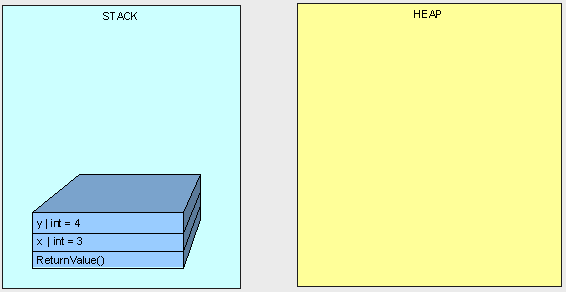1 public int ReturnValue2()
2 {
3 MyInt x;
4 x.MyValue = 3;
5 MyInt y;
6 y = x;
7 y.MyValue = 4;
8 return x.MyValue;
9 }